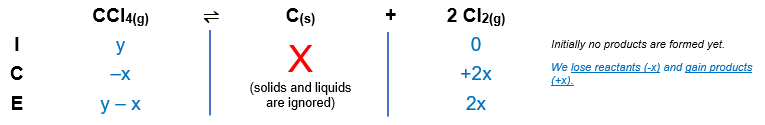# Problem: At 700 K, CCl4 decomposes to carbon and chlorine. The Kp for the decomposition is 0.76. Find the starting pressure of CCl4 at this temperature that produces a total pressure of 1.6 atm at equilibrium.

###### FREE Expert Solution

Reaction:         CCl4(g) ⇌ C(s) + 2 Cl2(g)             Kp = 0.76

Step 1: Construct an ICE chart for the equilibrium reaction.

Let y = initial pressure of CCl4Step 2: Calculate the change (x) in the reaction using the equilibrium constant.

(y – x) + 2x = total pressure

– x + 2x = 1.6

y + x = 1.6

y = 1.6 x

$\overline{){{\mathbf{K}}}_{{\mathbf{p}}}{\mathbf{=}}\frac{\mathbf{products}}{\mathbf{reactants}}}\phantom{\rule{0ex}{0ex}}{\mathbf{K}}_{\mathbf{p}}\mathbf{=}\frac{{\left({P}_{{\mathrm{Cl}}_{2}}\right)}^{\mathbf{2}}}{{\mathbf{P}}_{{\mathbf{CCl}}_{\mathbf{4}}}}\phantom{\rule{0ex}{0ex}}\mathbf{0}\mathbf{.}\mathbf{76}\mathbf{=}\frac{{\left(2x\right)}^{\mathbf{2}}}{\mathbf{y}\mathbf{-}\mathbf{x}}\phantom{\rule{0ex}{0ex}}\mathbf{0}\mathbf{.}\mathbf{76}\mathbf{=}\frac{{\left(2x\right)}^{2}}{\left(1.6-x\right)\mathbf{-}\mathbf{x}}\phantom{\rule{0ex}{0ex}}\mathbf{0}\mathbf{.}\mathbf{76}\left(1.6-2x\right)\mathbf{=}\frac{4{x}^{2}}{\overline{)1.6-2x}}\left(\overline{)1.6-2x}\right)\phantom{\rule{0ex}{0ex}}\mathbf{1}\mathbf{.}\mathbf{216}\mathbf{-}\mathbf{1}\mathbf{.}\mathbf{52}\mathbf{x}\mathbf{=}\mathbf{4}{\mathbf{x}}^{\mathbf{2}}\phantom{\rule{0ex}{0ex}}\overline{)\mathbf{0}\mathbf{=}\mathbf{4}{\mathbf{x}}^{\mathbf{2}}\mathbf{+}\mathbf{1}\mathbf{.}\mathbf{52}\mathbf{x}\mathbf{-}\mathbf{1}\mathbf{.}\mathbf{216}}$

Now, we will use the quadratic formula to solve for x. The quadratic formula is:

$\overline{){\mathrm{x}}{=}\frac{-\mathrm{b}±\sqrt{{\mathrm{b}}^{2}-4\mathrm{ac}}}{2\mathrm{a}}}$

Solving for x:

$\mathbf{x}\mathbf{=}\frac{\mathbf{-}\mathbf{\left(}\mathbf{1}\mathbf{.}\mathbf{52}\mathbf{\right)}\mathbf{±}\sqrt{\mathbf{\left(}\mathbf{1}\mathbf{.}\mathbf{52}{\mathbf{\right)}}^{\mathbf{2}}\mathbf{-}\mathbf{4}\mathbf{\left(}\mathbf{4}\mathbf{\right)}\mathbf{\left(}\mathbf{-}\mathbf{1}\mathbf{.}\mathbf{216}\mathbf{\right)}}}{\mathbf{2}\mathbf{\left(}\mathbf{4}\mathbf{\right)}}\phantom{\rule{0ex}{0ex}}\mathbf{x}\mathbf{=}\frac{\mathbf{-}\mathbf{1}\mathbf{.}\mathbf{52}\mathbf{±}\sqrt{\mathbf{2}\mathbf{.}\mathbf{3104}\mathbf{+}\mathbf{19}\mathbf{.}\mathbf{456}}}{\mathbf{8}}\phantom{\rule{0ex}{0ex}}\mathbf{x}\mathbf{=}\frac{\mathbf{-}\mathbf{1}\mathbf{.}\mathbf{52}\mathbf{±}\sqrt{\mathbf{21}\mathbf{.}\mathbf{7664}}}{\mathbf{8}}\phantom{\rule{0ex}{0ex}}\mathbf{x}\mathbf{=}\frac{\mathbf{-}\mathbf{1}\mathbf{.}\mathbf{52}\mathbf{±}\mathbf{4}\mathbf{.}\mathbf{66}}{\mathbf{8}}$

The calculation then splits into two ways:

80% (292 ratings)###### Problem Details

At 700 K, CCl4 decomposes to carbon and chlorine. The Kp for the decomposition is 0.76. Find the starting pressure of CCl4 at this temperature that produces a total pressure of 1.6 atm at equilibrium.

Frequently Asked Questions

What scientific concept do you need to know in order to solve this problem?

Our tutors have indicated that to solve this problem you will need to apply the ICE Chart concept. You can view video lessons to learn ICE Chart. Or if you need more ICE Chart practice, you can also practice ICE Chart practice problems.

What professor is this problem relevant for?

Based on our data, we think this problem is relevant for Professor Ensminger's class at COLUMBUS STATE.